# Linux seq Command Tutorial for Beginners (5 Examples)

Sometimes, you come across a command line tool that offers limited functionality on its own, but when used with other tools, you realize its actual potential. Once such tool is seq, which prints a sequence of numbers. In this tutorial, we will discuss the basics of this command line utility using easy to understand examples.

But before jumping on to that, it's worth mentioning that all examples in this article have been tested on an Ubuntu 16.04 machine.

## Linux seq command

As already mentioned, the seq command lets you print a sequence of numbers. Following is its syntax:

``seq [OPTION]... LASTseq [OPTION]... FIRST LASTseq [OPTION]... FIRST INCREMENT LAST``

And here's how the tool's man page explains it:

``       Print numbers from FIRST to LAST, in steps of INCREMENT. If FIRST or        INCREMENT is omitted, it defaults to 1.  That is, an omitted  INCREMENT        defaults to 1 even when LAST is smaller than FIRST.                The sequence of numbers ends when the sum of the current number and         INCREMENT  would  become  greater than LAST.  FIRST, INCREMENT, and LAST        are interpreted as floating point values.  INCREMENT is usually positive if       FIRST  is smaller than LAST, and INCREMENT is usually negative if FIRST       is greater than LAST.  FORMAT must be suitable for printing  one  argu?       ment  of type 'double'; it defaults to %.PRECf if FIRST, INCREMENT, and       LAST are all fixed point decimal numbers with maximum  precision  PREC,       and to %g otherwise.``

Following are some Q&A-styled examples that should give you a better idea on how the seq command works.

## Q1. How seq command works?

Basic usage is very easy. All you have to do is to pass a number to seq, and the tool will producein output numbers from 1 to the input number.

For example:

``seq 8``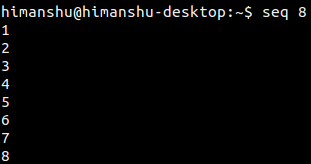Of course, you can also specify the number from which you want the output to begin with.

For example:

``seq 3 8``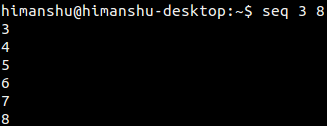Movin on, you can also set the incremental difference, which is 1 by default. For example, if you want seq to print from 1 to 9, but with a difference of 2, then here's how you can do that:

``seq 1 2 9``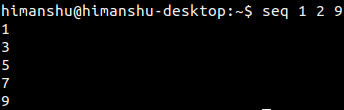## Q2. How to add a separator?

If you want, you can also have a separator to make the seq output look better. This feature is available through the -s command line option.

For example, the following command intends to use a comma (,) as a separator:

``seq -s, 1 9``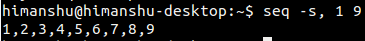## Q3. How to specify output format?

The seq command allows you to use printf style floating-point FORMAT. This feature is accessible through the -f command line option. The tool's man page doesn't have much information on how to use this option, but the info page contains the required details. Here's what the info page says:

```-f FORMAT'`--format=FORMAT' Print all numbers using FORMAT. FORMAT must contain exactly one of the `printf'-style floating point conversion specifications `%a', `%e', `%f', `%g', `%A', `%E', `%F', `%G'. The `%' may be followed by zero or more flags taken from the set `-+#0 '', then an optional width containing one or more digits, then an optional precision consisting of a `.' followed by zero or more digits. FORMAT may also contain any number of `%%' conversion specifications. All conversion specifications have the same meaning as with `printf'. The default format is derived from FIRST, STEP, and LAST. If these all use a fixed point decimal representation, the default format is `%.Pf', where P is the minimum precision that can represent the output numbers exactly. Otherwise, the default format is `%g'.``

For example, you can use this option in the following way:

``seq -f "%02g" 6``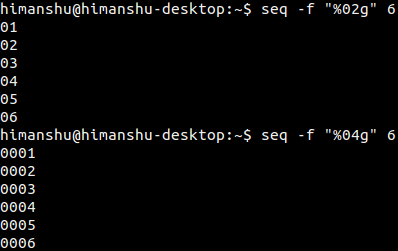## Q4. How to use seq with other commands? (Use case 1)

Suppose you want to perform addition of some numbers, say from 111 to 121. Here's how you can do this using seq:

``expr `seq -s " + " 111 121```

Here's the above command in action: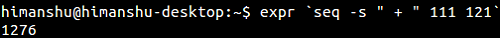## Q5. How to use seq with other commands? (Use case 2)

Suppose you want to create a bunch of new files with names where only an integer value changes. For example, file1, file2, file3, and so on. Here's how you can do this using seq.

touch \$(seq -f "file%g" 1 10)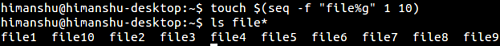## Conclusion

So now you'll agree how useful the seq command is. If you talk about the command line options Seq offers, there's not much of a learning curve, just that you should know when and how to use the command and its options. We've covered several use cases here - should be enough to get you started with the tool. For more information on Seq, head to its man page.view as pdf |print

## 3 Comment(s)

Add comment

### Comments

By: thecursedfly

Small mistake I guess:

"Suppose you want to perform addition of some numbers, say from 1 to 10." should be "from 111 to 121".

By: guest

seq -s " + " 111 121

in my shell creates "111 + 112 + 113 + 114 + 115 + 116 + 117 + 118 + 119 + 120 + 121 +"

and "expr" will then complain (syntax error) for the last +

A possible fix is    seq -s " + " -t 0 111 121

By: Holgi

you could even use both -format and --separator in one command:

seq -f "'%g'" -s "," 90 111

'90','91','92','93','94','95','96','97','98','99','100','101','102','103','104','105','106','107','108','109','110','111'